# NCERT solutions for Mathematics Exemplar Class 9 chapter 12 - Heron's Formula [Latest edition]

#### Chapters## Chapter 12: Heron's Formula

Exercise 12.1Exercise 12.2Exercise 12.3Exercise 12.4
Exercise 12.1 [Pages 113 - 114]

### NCERT solutions for Mathematics Exemplar Class 9 Chapter 12 Heron's Formula Exercise 12.1 [Pages 113 - 114]

#### State whether the following statement is True or False:

Exercise 12.1 | Q 1 | Page 113

An isosceles right triangle has area 8 cm2. The length of its hypotenuse is ______.

• sqrt(32) cm

• sqrt(16) cm

• sqrt(48) cm

• sqrt(24) cm

Exercise 12.1 | Q 2 | Page 114

The perimeter of an equilateral triangle is 60 m. The area is ______.

• 10sqrt(3) cm2

• 15sqrt(3) cm2

• 20sqrt(3) cm2

• 100sqrt(3) cm2

Exercise 12.1 | Q 3 | Page 114

The sides of a triangle are 56 cm, 60 cm and 52 cm long. Then the area of the triangle is ______.

• 1322 cm2

• 1311 cm2

• 1344 cm2

• 1392 cm

Exercise 12.1 | Q 4 | Page 114

The area of an equilateral triangle with side 2sqrt(3) cm is ______.

• 5.196 cm

• 0.866 cm2

• 3.496 cm2

• 1.732 cm2

Exercise 12.1 | Q 5 | Page 114

The length of each side of an equilateral triangle having an area of 9sqrt(3)cm2 is ______.

• 8 cm

• 36 cm

• 4 cm

• 6 cm

Exercise 12.1 | Q 6 | Page 114

If the area of an equilateral triangle is 16sqrt(3) cm2, then the perimeter of the triangle is ______.

• 48 cm

• 24 cm

• 12 cm

• 36 cm

Exercise 12.1 | Q 7 | Page 114

The sides of a triangle are 35 cm, 54 cm and 61 cm, respectively. The length of its longest altitude ______.

• 16sqrt(5) cm

• 10sqrt(5) cm

• 24sqrt(5) cm

• 28 cm

Exercise 12.1 | Q 8 | Page 114

The area of an isosceles triangle having base 2 cm and the length of one of the equal sides 4 cm, is ______.

• sqrt(15) cm2

• sqrt(15/2) cm2

• 2sqrt(15) cm2

• 4sqrt(15) cm2

Exercise 12.1 | Q 9 | Page 114

The edges of a triangular board are 6 cm, 8 cm and 10 cm. The cost of painting it at the rate of 9 paise per cm2 is ______.

• Rs 2.00

• Rs 2.16

• Rs 2.48

• Rs 3.00

Exercise 12.2 [Page 115]

### NCERT solutions for Mathematics Exemplar Class 9 Chapter 12 Heron's Formula Exercise 12.2 [Page 115]

#### State whether the following statement is True or False:

Exercise 12.2 | Q 1 | Page 115

The area of a triangle with base 4 cm and height 6 cm is 24 cm2.

• True

• False

Exercise 12.2 | Q 2 | Page 115

The area of ∆ABC is 8 cm2 in which AB = AC = 4 cm and ∠A = 90º.

• True

• False

Exercise 12.2 | Q 3 | Page 115

The area of the isosceles triangle is 5/4 sqrt(11) cm2, if the perimeter is 11 cm and the base is 5 cm.

• True

• False

Exercise 12.2 | Q 4 | Page 115

The area of the equilateral triangle is 20sqrt(3) cm2 whose each side is 8 cm.

• True

• False

Exercise 12.2 | Q 5 | Page 115

If the side of a rhombus is 10 cm and one diagonal is 16 cm, the area of the rhombus is 96 cm2.

• True

• False

Exercise 12.2 | Q 6 | Page 115

The base and the corresponding altitude of a parallelogram are 10 cm and 3.5 cm, respectively. The area of the parallelogram is 30 cm2.

• True

• False

Exercise 12.2 | Q 7 | Page 115

The area of a regular hexagon of side ‘a’ is the sum of the areas of the five equilateral triangles with side a.

• True

• False

Exercise 12.2 | Q 8 | Page 115

The cost of levelling the ground in the form of a triangle having the sides 51 m, 37 m and 20 m at the rate of Rs 3 per m2 is Rs 918.

• True

• False

Exercise 12.2 | Q 9 | Page 115

In a triangle, the sides are given as 11 cm, 12 cm and 13 cm. The length of the altitude is 10.25 cm corresponding to the side having length 12 cm.

• True

• False

Exercise 12.3 [Page 117]

### NCERT solutions for Mathematics Exemplar Class 9 Chapter 12 Heron's Formula Exercise 12.3 [Page 117]

Exercise 12.3 | Q 1 | Page 117

Find the cost of laying grass in a triangular field of sides 50 m, 65 m and 65 m at the rate of Rs 7 per m2.

Exercise 12.3 | Q 2 | Page 117

The triangular side walls of a flyover have been used for advertisements. The sides of the walls are 13 m, 14 m and 15 m. The advertisements yield an earning of Rs 2000 per m2 a year. A company hired one of its walls for 6 months. How much rent did it pay?

Exercise 12.3 | Q 3 | Page 117

From a point in the interior of an equilateral triangle, perpendiculars are drawn on the three sides. The lengths of the perpendiculars are 14 cm, 10 cm and 6 cm. Find the area of the triangle.

Exercise 12.3 | Q 4 | Page 117

The perimeter of an isosceles triangle is 32 cm. The ratio of the equal side to its base is 3:2. Find the area of the triangle.

Exercise 12.3 | Q 5 | Page 117

Find the area of a parallelogram given in figure. Also find the length of the altitude from vertex A on the side DC.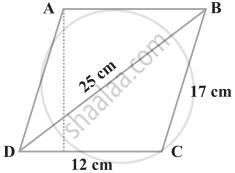Exercise 12.3 | Q 6 | Page 117

A field in the form of a parallelogram has sides 60 m and 40 m and one of its diagonals is 80 m long. Find the area of the parallelogram.

Exercise 12.3 | Q 7 | Page 117

The perimeter of a triangular field is 420 m and its sides are in the ratio 6:7:8. Find the area of the triangular field.

Exercise 12.3 | Q 8 | Page 117

The sides of a quadrilateral ABCD are 6 cm, 8 cm, 12 cm and 14 cm (taken in order) respectively, and the angle between the first two sides is a right angle. Find its area.

Exercise 12.3 | Q 9 | Page 117

A rhombus shaped sheet with perimeter 40 cm and one diagonal 12 cm, is painted on both sides at the rate of Rs 5 per m2 . Find the cost of painting.

Exercise 12.3 | Q 10 | Page 117

Find the area of the trapezium PQRS with height PQ given in figure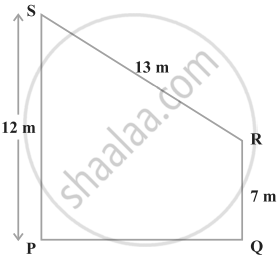Exercise 12.4 [Pages 118 - 120]

### NCERT solutions for Mathematics Exemplar Class 9 Chapter 12 Heron's Formula Exercise 12.4 [Pages 118 - 120]

Exercise 12.4 | Q 1 | Page 118

How much paper of each shade is needed to make a kite given in figure, in which ABCD is a square with diagonal 44 cm.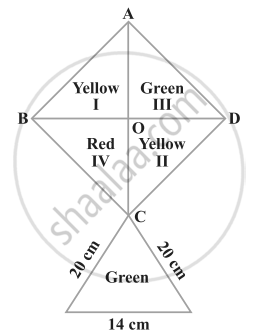Exercise 12.4 | Q 2 | Page 119

The perimeter of a triangle is 50 cm. One side of a triangle is 4 cm longer than the smaller side and the third side is 6 cm less than twice the smaller side. Find the area of the triangle.

Exercise 12.4 | Q 3 | Page 119

The area of a trapezium is 475 cm2 and the height is 19 cm. Find the lengths of its two parallel sides if one side is 4 cm greater than the other.

Exercise 12.4 | Q 4 | Page 119

A rectangular plot is given for constructing a house, having a measurement of 40 m long and 15 m in the front. According to the laws, a minimum of 3 m, wide space should be left in the front and back each and 2 m wide space on each of other sides. Find the largest area where house can be constructed.

Exercise 12.4 | Q 5 | Page 119

A field is in the shape of a trapezium having parallel sides 90 m and 30 m. These sides meet the third side at right angles. The length of the fourth side is 100 m. If it costs Rs 4 to plough 1 m2 of the field, find the total cost of ploughing the field.

Exercise 12.4 | Q 6 | Page 119

In figure, ∆ ABC has sides AB = 7.5 cm, AC = 6.5 cm and BC = 7 cm. On base BC a parallelogram DBCE of same area as that of ∆ABC is constructed. Find the height DF of the parallelogram.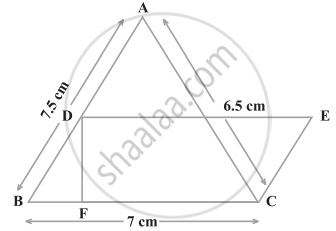Exercise 12.4 | Q 7 | Page 119

The dimensions of a rectangle ABCD are 51 cm × 25 cm. A trapezium PQCD with its parallel sides QC and PD in the ratio 9:8, is cut off from the rectangle as shown in the figure. If the area of the trapezium PQCD is 5/6 h part of the area of the rectangle, find the lengths QC and PD.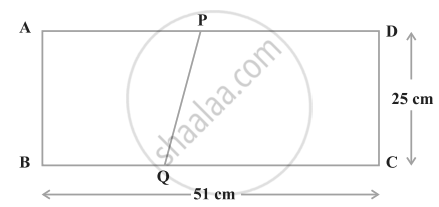Exercise 12.4 | Q 8 | Page 120

A design is made on a rectangular tile of dimensions 50 cm × 70 cm as shown in figure. The design shows 8 triangles, each of sides 26 cm, 17 cm and 25 cm. Find the total area of the design and the remaining area of the tile.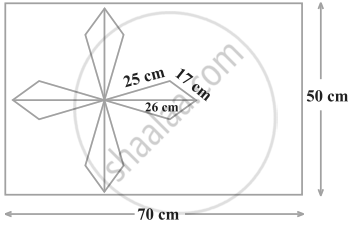## Chapter 12: Heron's Formula

Exercise 12.1Exercise 12.2Exercise 12.3Exercise 12.4## NCERT solutions for Mathematics Exemplar Class 9 chapter 12 - Heron's Formula

NCERT solutions for Mathematics Exemplar Class 9 chapter 12 (Heron's Formula) include all questions with solution and detail explanation. This will clear students doubts about any question and improve application skills while preparing for board exams. The detailed, step-by-step solutions will help you understand the concepts better and clear your confusions, if any. Shaalaa.com has the CBSE Mathematics Exemplar Class 9 solutions in a manner that help students grasp basic concepts better and faster.

Further, we at Shaalaa.com provide such solutions so that students can prepare for written exams. NCERT textbook solutions can be a core help for self-study and acts as a perfect self-help guidance for students.

Concepts covered in Mathematics Exemplar Class 9 chapter 12 Heron's Formula are Area of a Triangle by Heron's Formula, Application of Heron’s Formula in Finding Areas of Quadrilaterals, Area of a Triangle.

Using NCERT Class 9 solutions Heron's Formula exercise by students are an easy way to prepare for the exams, as they involve solutions arranged chapter-wise also page wise. The questions involved in NCERT Solutions are important questions that can be asked in the final exam. Maximum students of CBSE Class 9 prefer NCERT Textbook Solutions to score more in exam.

Get the free view of chapter 12 Heron's Formula Class 9 extra questions for Mathematics Exemplar Class 9 and can use Shaalaa.com to keep it handy for your exam preparation